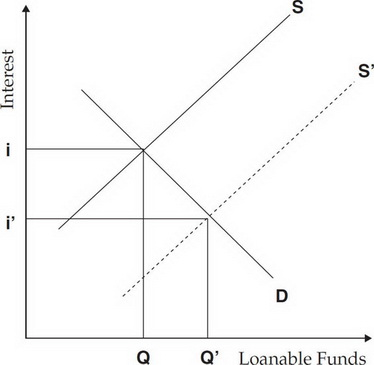# AP Macroeconomics Question 4: Answer and Explanation

### Test Information

Question: 4

4. A financial planner on a popular TV show convinces more Americans to save for retirement. What is the result on the supply and demand for loanable funds?

• A. The supply curve would shift up, increasing the equilibrium interest rate.
• B. The demand curve would shift up, increasing the equilibrium interest rate.
• C. The supply curve would shift down, decreasing the equilibrium interest rate.
• D. The demand curve would shift down, decreasing the equilibrium interest rate.
• E. Both the supply and demand curves would shift.